# I tried using the Android version of "PhotoMath" that will show you not only the answer but also the solution, when taking a mathematical expression with a smartphone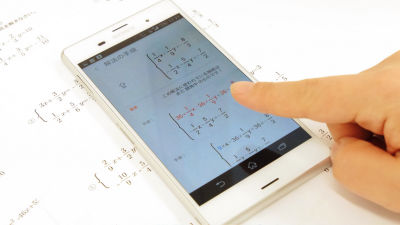A free application "PhotoMath" that automatically answers math problems with smartphones with answers on the wayInitially, only the iOS application was released, but the Android application was released on March 2, 2015. Also, what was only supported for "arithmetic expression" "fractional / fractional number" "roots" "linear equation" was newly expressed as "quadratic equation" "inequality" "simultaneous equations" "equation including absolute value sign" Since it is said that it became able to solve, etc, actually I tried using the Android application.

PhotoMath.net
https://photomath.net/en/

You can check how you actually use PhotoMath for Android from the following movie.

I tried using the Android version of PhotoMath which solves just by scanning the formula - YouTube

Install the Android application from the following website.

PhotoMath - Android Apps on Google Play

Install first.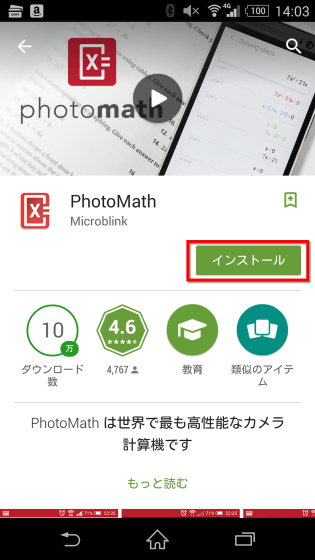Tap "I agree".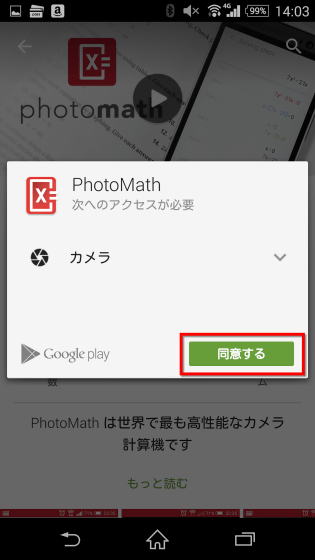Tap "Open" when the application is installed.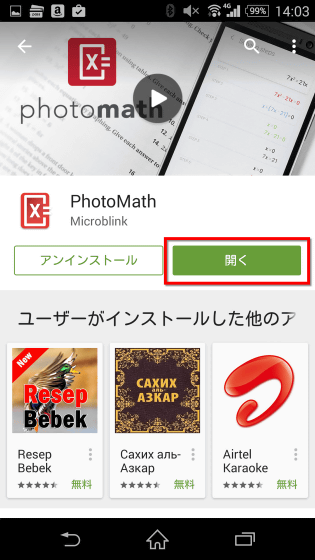Then the following screen will be displayed. The person who used it for the first time was able to see the instruction by tapping "go". So tap "Go".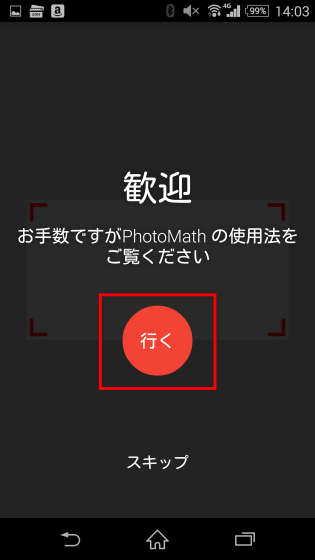How to use PhotoMath is very easy, just put a formula in the red frame on the screen.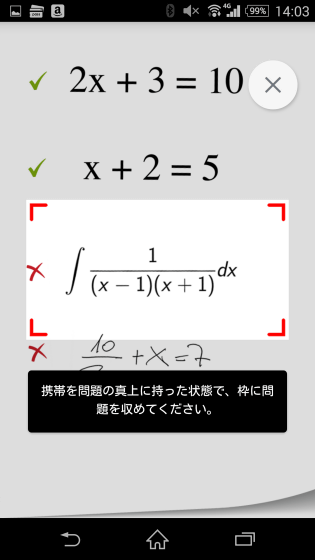If you adjust the size of the red frame so that only the kitchen formula is included in the red frame, the application will calculate it without permission and calculate the answer without permission, afterwards.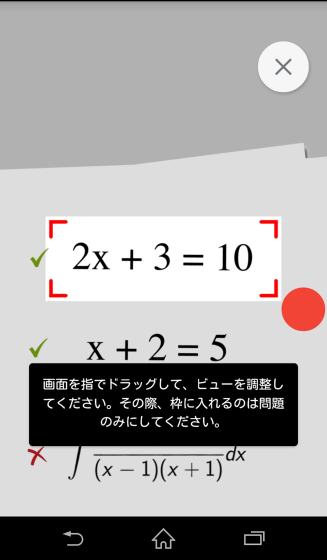Some of the mathematical expressions are complicated, which applications are not yet supported.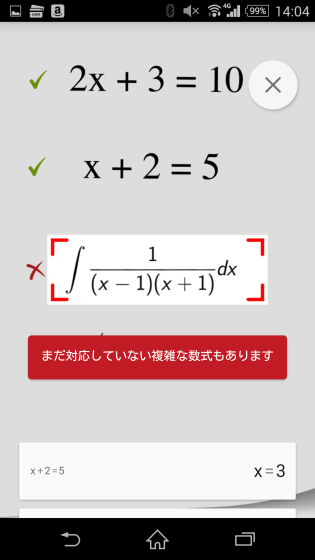In addition, it does not correspond to handwritten characters.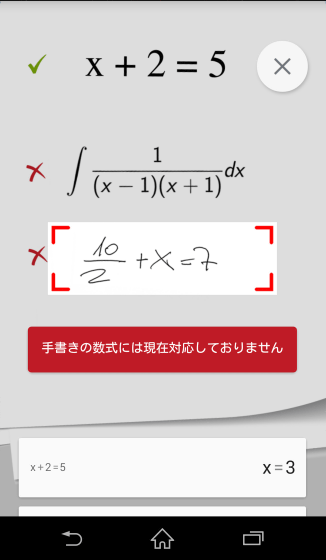That is why I actually use the application from below. The formula isMathematics Ⅰ Equations and exercises on inequality simultaneous inequalitiesWe have used simultaneous inequalities.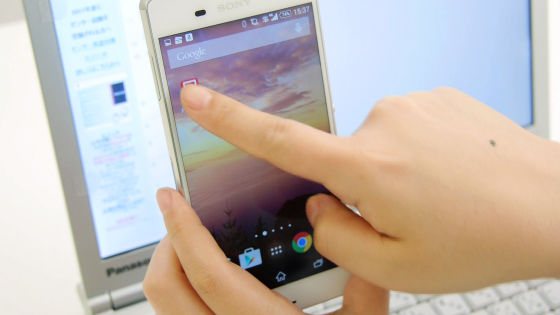When you launch the application, the following screen is displayed, so first adjust the size of the red frame to match the formula you want to read.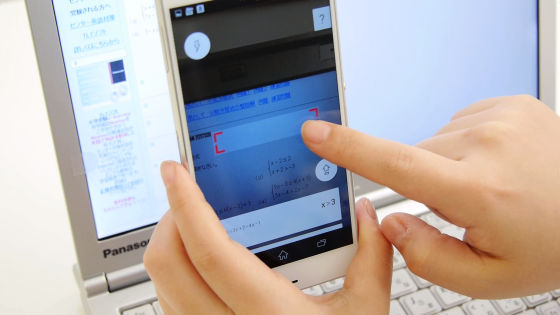If you put only the kitchen formula in the red frame, the answer was displayed under the formula in a blink of an eye in a blink of an eye.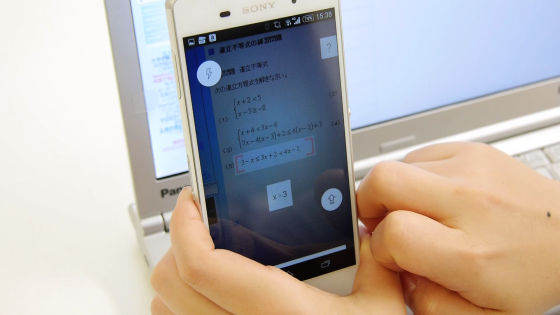Furthermore, a log of "Recently Scanned Formula" appears at the bottom of the screen, so tap it.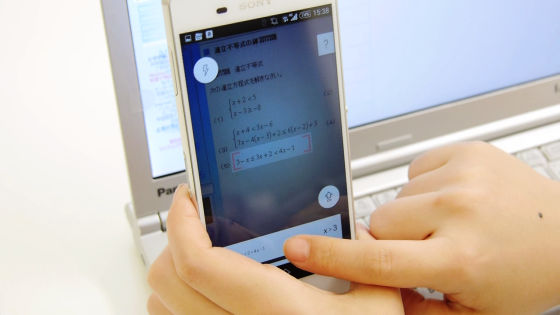As shown below, the solution was shown carefully.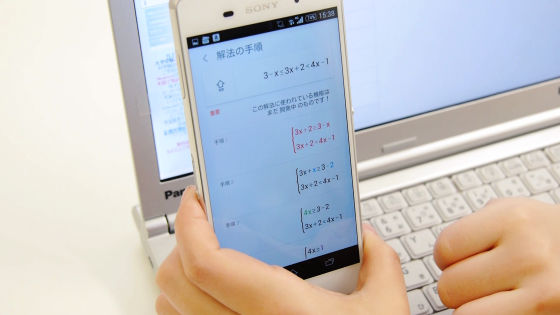The solution is displayed in multiple steps and it is possible to read further explanation by tapping each procedure.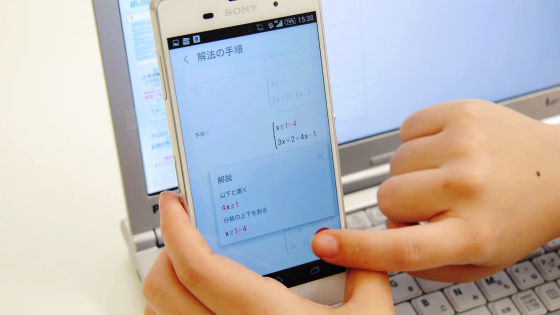When I tried it further, I could read simultaneous equations using fractions.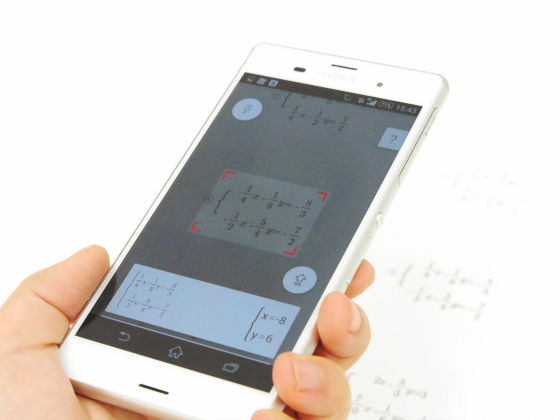In the procedure of the solution method, although the indication "The function used in this solution is still under development!" Appears, the correct answer was derived.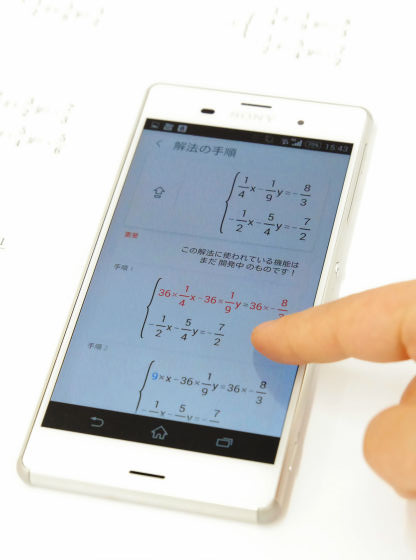It is also possible to solve quadratic equations.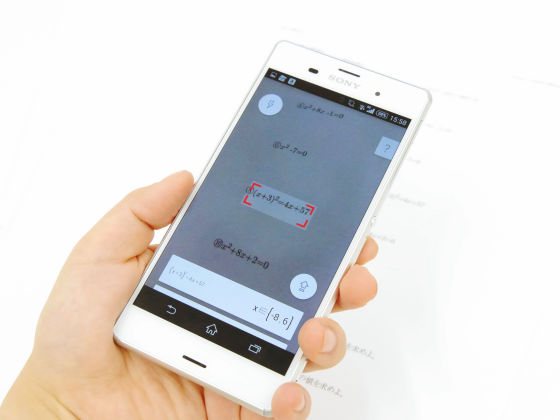At the time the application was released, I could only solve a simple linear equation, but it is an impression that it corresponds to quadratic equations, simultaneous equations, etc. and it evolved steadily. If there are numbers etc. at the beginning of the problem, we could not fit just the mathematics in the red frame and sometimes took a bit of trouble, but it's quite usable because we do not need to enter the numbers. Of course, there are complicated mathematical formulas that are not yet supported, but if you are watching this update, it seems that you will be able to expect sufficient improvements in future applications.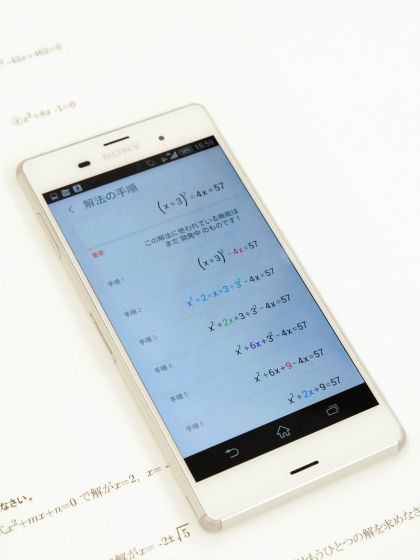Currently PhotoMath isWindows StoreYaAmazonYou can install from.

in Review,   Software,   Video, Posted by logq_fa